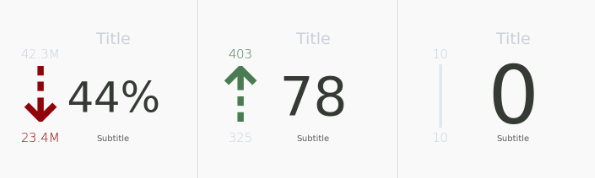# Change in KPI ChartMotivation

A frequent business question often posted is "What is the change in [users, units sold, views, etc]? Using Python's matplotlib library, one way to visualize this change is to visualize it alongside an arrow indicating the direction of change along with the context provided by the addition of the previous and current metric.

SQL Data Input Format

A dataframe with two rows with the first column containing the metrics to be visualized. The df is ordered by date descending so the first row relates to the most recent metric and the second row relates to the previous metric.

Python Chart Script

import pandas as pd
import numpy as np
import matplotlib.pyplot as plt
import matplotlib.path as mpath
import matplotlib.lines as mlines
import matplotlib.patches as mpatches
from matplotlib.collections import PatchCollection

##########################################################################
### HELPER UTILITY FUNCTIONS
##########################################################################

# Function: Takes an integer/float and returns a string with the number rounded to the nearest thousand place and appends the appropriate thousand's place symbol
# Input: float/intger
# Output: Formatted string
def prettify_num(num):
pretty_num = ''
if abs(num) < 10**3:
pretty_num = str(num)
elif abs(num) < 10**6:
pretty_num = str(round(1.0*num/10**3,1)) + 'K'
elif abs(num) < 10**9:
pretty_num = str(round(1.0*num/10**6,1)) + 'M'
else:
pretty_num = str(round(1.0*num/10**9,1)) + 'B'
return pretty_num

def color_difference(first, second):
if first > second:
return '#8b0000' #red
elif second > first:
return '#4f7c4f' #green
else: return '#e3e7ed' #gray

# Function: Parse the df and returns the numbers contextualized
# Input: df with at least two columns
# Output: an array of three numeric or null values: the percent(0-1), the current number, and the contract number

def parse(df):
if df.size == 0:
return [None, None]
elif df.iloc[0,0] == -1:
current = pd.to_numeric(df.iloc[0,1])
return [current, np.inf]
else:
past = pd.to_numeric(df.iloc[1,0])
current = pd.to_numeric(df.iloc[0,0])
return [past, current]

# Function: Parse the df and returns the prettified numbers to be sent to the matplot plot
# Input: df with at least two columns
# Output: an array of three prettified strings: the percent, the current number, and the contract number
def pretty_parse(df):
[current, contract] = parse(df)
if df.size == 0:
return ['', '', 'NA']
elif contract == np.inf:
current = str(prettify_num(current))
return [current, '$\infty$', '$\infty$']
else:
return [str(prettify_num(current)),
str(prettify_num(contract)),
str(prettify_num(current-contract)),
pretty_percent(current, contract)]

##########################################################################
### HELPER PLOT FUNCTIONS
##########################################################################

# Function: create a centered text object
# Input: the axes object to append to and optionally (text, color, y_position, font_size)
# Output: matplot ax.text object
def kpi_text(ax, text = '', text_color = 'black', y_position = 0, font_size = 10):
return ax.text(x = 0.5,
y = y_position,
s = text,
color = text_color,
family = 'sans-serif',
fontsize = font_size,
fontweight = 500,
horizontalalignment = 'center',
verticalalignment = 'center')

def get_arrow(past, current):
if past > current:
return '⇣'
elif current > past:
return '⇡'
else:
return '|'

def get_main_font_size(main_text, type):
if type == 'percent':
if len(main_text) > 3:
return 80
return 100
else:
if len(main_text) > 5:
return 65
else:
return 80

##########################################################################
### MAIN PLOT
##########################################################################

def kpi_chart(df, titletext = 'KPI', subtitle = '', type = 'absolute'):

# Parse data
[past, current] = parse(df)
diff = abs(current - past)
percent = 1.0 * (current-past)/past if df.size != 0 else None
[ppast, pcurrent, pdiff] = map(prettify_num, [past, current, diff])

# Set up figure canvas
fig, ax = plt.subplots(figsize = (5,5))
fig.subplots_adjust(left=0, bottom=0, right=1, top=1, wspace=0, hspace=0)
ax.axis('off')

#Colors
mediumgray = '#999292'
lightgray = '#cbd0d8'
darkergreen = '#4f7c4f'
lightergreen = '#639b63'
darkred = '#a60c3a'

ppercent = str(abs(int(percent*100))) + '%'
main_text = ppercent if type == 'percent' else prettify_num(diff)

# Font Size
arrow_position_x = 0.2
main_position_x = 0.68
main_text_y = 0.45
label_text_y = main_text_y - 0.25

title = ax.text(x = main_position_x,
y = 0.85,
s = titletext,
color = lightgray,
family = 'sans-serif',
fontsize = 38,
fontweight = 450,
horizontalalignment = 'center',
verticalalignment = 'center')

arrow = ax.text(x = arrow_position_x,
y = main_text_y if past != current else main_text_y + 0.03,
s = get_arrow(past, current),
color = color_difference(past, current),
family = 'sans-serif',
fontsize = 180 if past != current else 150,
fontweight = 50 if past == current else 300,
horizontalalignment = 'center',
verticalalignment = 'center')

main = ax.text(x = main_position_x,
y = main_text_y,
s = main_text,
color = '#353a34',
family = 'sans-serif',
fontsize = get_main_font_size(main_text, type),
#                    fontsize = 380 / (len(main_text)) if type == 'absolute' else 300/len(main_text),
fontweight = 500,
horizontalalignment = 'center',
verticalalignment = 'center')

label = ax.text(x = main_position_x,
y = label_text_y,
s = subtitle,
color = '#595a5b',
family = 'sans-serif',
fontsize = 20,
fontweight = 450,
horizontalalignment = 'center',
verticalalignment = 'center')

top = ax.text(x = arrow_position_x,
y = 0.75,
s = pcurrent if current > past else ppast,
family = 'sans-serif',
fontsize = 30,
fontweight = 50,
color = color_difference(past, current) if current > past else lightgray,
horizontalalignment = 'center',
verticalalignment = 'center')

bottom = ax.text(x = arrow_position_x,
y = 0.2,
s = pcurrent if current < past else ppast,
family = 'sans-serif',
fontsize = 30,
fontweight = 50,
color = color_difference(past, current) if current < past else lightgray,
horizontalalignment = 'center',
verticalalignment = 'center')

return ax

periscope.output(kpi_chart(df, titletext = 'Title', subtitle = 'Subtitle', type = 'percent'))